# 论文解读（ N2N）《Node Representation Learning in Graph via Node-to-Neighbourhood Mutual Information Maximization》

2022-04-01 16:30 1611 查看

# 1 摘要

在本工作中，我们提出了一种简单而有效的自监督节点表示学习策略，通过直接最大化节点的隐藏表示及其邻域之间的互信息，这可以通过图平滑理论证明。在 InfoNCE 之后，我们的框架通过一个替代对比损失进行了优化，其中正样本选择支撑了表示学习的质量和效率。为此，我们提出了一种拓扑感知的正采样策略，该策略通过考虑节点之间的结构依赖性，从邻域进行正采样，从而能够提前进行正选择。在极端情况下，当只有一个正的采样时，我们完全避免了昂贵的邻域聚合。我们的方法在各种节点分类数据集上都取得了很好的性能。值得一提的是，通过将我们的损失函数应用于基于 MLP 的节点编码器，我们的方法可以比现有的解决方案更快。

# 2 介绍

本文任务：节点分类。[ 关键：通过从邻域获取上下文信息来学习带结构信息的节点表示 ]

GNNs 过程可总结为： Aggregation-Combine-Prediction pipeline

Aggregation 步骤通过 mean 、max 、attention  和 ensemble  等各种邻域聚合器将邻域信息聚合为向量化表示，并通过 sum 或 concatenation 与节点表示相结合，实现邻域信息融合。为获得多跳信息，在最终获得用于预测节点标签的表示之前，常重复采用 Aggregation-Combine 操作。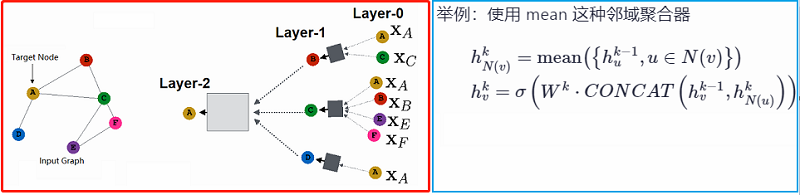# 3 方法

## 3.1 GNN 框架

有监督的GNN 框架为：

$\begin{array}{l}\overrightarrow{\boldsymbol{s}}_{i}^{(l-1)} &=\operatorname{AGGREGATION}\left(\left\{\overrightarrow{\boldsymbol{h}}_{j}^{(l-1)}: v_{j} \in \mathcal{N}_{i}\right\}\right) \\\overrightarrow{\boldsymbol{h}}_{i}^{(l)} &=\operatorname{COMBINE}\left(\left\{\overrightarrow{\boldsymbol{s}}_{i}^{(l-1)}, \overrightarrow{\boldsymbol{h}}_{i}^{(l-1)}\right\}\right) \\\mathcal{L}_{\mathrm{CE}} &=\operatorname {PREDICTION}\left(\left\{\overrightarrow{\boldsymbol{h}}_{i}^{(L)}, y_{v_{i}}\right\}\right)\end{array}\quad\quad\quad\quad(1)$

## 3.2.节点到邻域（N2N）互信息最大化

基于特征空间 $\mathcal{X}^{D^{(l)}}$的节点表示 $\overrightarrow{\boldsymbol{h}}_{i}^{(l)}$ 的概率密度函数 $p\left(H(\boldsymbol{x})^{(l)}\right)$，同样邻居节点表示也类似$p\left(S(\boldsymbol{x})^{(l)}\right)$，我们将节点表示与其对应的邻域表示之间的互信息定义为：

$I\left(S(\boldsymbol{x})^{(l)} ; H(\boldsymbol{x})^{(l)}\right)= \int_{\mathcal{X}^{(l)}} p\left(S(\boldsymbol{x})^{(l)}, H(\boldsymbol{x})^{(l)}\right) \cdot \log \frac{p\left(S(\boldsymbol{x})^{(l)}, H(\boldsymbol{x})^{(l)}\right)}{p\left(S(\boldsymbol{x})^{(l)}\right) \cdot p\left(H(\boldsymbol{x})^{(l)}\right)} d \boldsymbol{x}\quad\quad\quad\quad(2)$

PS：互信息  $I(X ; Y)$  是联合分布  $p(x, y)$ 与 边缘分布  $p(x) p(y)$  的相对熵。

互信息难计算的原因：由于在连续和高维空间中。

幸运的是，通过 Mutual Information Neural Estimation (MINE) ，可实现互信息计算，它将互信息最大化转化为最小化 $\text{InfoNCE}$ 损失，将 $\text{Eq. (2)}$ 中的 N2N 互信息损失转换为：

\begin{aligned}\mathcal{L}_{\text {InfoNCE }} =-\mathbb{E}_{v_{i} \in \mathcal{V}}\left[\log \frac{\exp \left(\operatorname{sim}\left(\overrightarrow{\boldsymbol{s}}_{i}^{(l)}, \overrightarrow{\boldsymbol{h}}_{i}^{(l)}\right) / \tau\right)}{\sum_{v_{k} \in \mathcal{V}} \exp \left(\operatorname{sim}\left(\overrightarrow{\boldsymbol{h}}_{k}^{(l)}, \overrightarrow{\boldsymbol{h}}_{i}^{(l)}\right) / \tau\right)}\right]\end{aligned}\quad\quad\quad(3)

最大化互信息 $I\left(S(\boldsymbol{x})^{(l)} ; H(\boldsymbol{x})^{(l)}\right)$ 起着图平滑的作用 ，这被证明对节点/图预测是积极的，在此，本文引入特征平滑度量：
$\delta_{f}^{(l)}=\frac{\left\|\sum_{v_{i} \in \mathcal{V}}\left(\sum_{v_{j} \in \mathcal{N}_{i}}\left(\overrightarrow{\boldsymbol{h}}_{i}^{(l)}-\overrightarrow{\boldsymbol{h}}_{j}^{(l)}\right)\right)^{2}\right\|_{1}}{|\mathcal{E}| \cdot D^{(l)}}\quad\quad\quad(4)$

工作[ 18 ] 进一步提出从邻居表示 $\overrightarrow{\boldsymbol{s}}_{i}^{(l)}$ 得到的信息，可以表达为 KL 散度的形式：

$D_{K L}\left(S(\boldsymbol{x})^{(l)} \| H(\boldsymbol{x})^{(l)}\right)= \int_{\mathcal{X}^{(l)}} p\left(S(\boldsymbol{x})^{(l)}\right) \cdot \log \frac{p\left(S(\boldsymbol{x})^{(l)}\right)}{p\left(H(\boldsymbol{x})^{(l)}\right)} d \boldsymbol{x}\quad\quad\quad(5)$

$\text{Eq. (5)}$ 和特征平滑度量有着很大的关联，即 $D_{K L}\left(S(\boldsymbol{x})^{(l)} \| H(\boldsymbol{x})^{(l)}\right) \sim \delta_{f}^{(l)}$。

通过互信息与信息熵之间的关系，我们得到：

$I\left(S(\boldsymbol{x})^{(l)} ; H(\boldsymbol{x})^{(l)}\right)= \mathrm{H}\left(S(\boldsymbol{x})^{(l)}\right)+\mathrm{H}\left(H(\boldsymbol{x})^{(l)}\right)-\mathrm{H}\left(S(\boldsymbol{x})^{(l)}, H(\boldsymbol{x})^{(l)}\right)\quad\quad\quad(10)$

其中：$\mathrm{H}(\cdot)$ 是信息熵，$\mathrm{H}(\cdot, \cdot)$ 是联合信息熵。

带信息熵的KL散度定义为：

$D_{K L}\left(S(\boldsymbol{x})^{(l)} \| H(\boldsymbol{x})^{(l)}\right)= \mathrm{H}\left(S(\boldsymbol{x})^{(l)}, H(\boldsymbol{x})^{(l)}\right)-\mathrm{H}\left(S(\boldsymbol{x})^{(l)}\right)\quad\quad\quad(11)$

应用 $\text{Eq.10}$ 和 $\text{Eq.11}$ 得到：

$\begin{array}{l} I\left(S(\boldsymbol{x})^{(l)} ; H(\boldsymbol{x})^{(l)}\right)&=\mathrm{H}\left(S(\boldsymbol{x})^{(l)}\right)+\mathrm{H}\left(H(\boldsymbol{x})^{(l)}\right) -D_{K L}\left(S(\boldsymbol{x})^{(l)} \| H(\boldsymbol{x})^{(l)}\right)-\mathrm{H}\left(S(\boldsymbol{x})^{(l)}\right) \\ &=\mathrm{H}\left(H(\boldsymbol{x})^{(l)}\right)-D_{K L}\left(S(\boldsymbol{x})^{(l)} \| H(\boldsymbol{x})^{(l)}\right) \end{array}\quad\quad\quad(12)$

由  $\text{Eq.12}$ 便得到结论：

${\large I\left(S(\boldsymbol{x})^{(l)} ; H(\boldsymbol{x})^{(l)}\right) \sim \frac{1}{D_{K L}\left(S(\boldsymbol{x})^{(l)} \| H(\boldsymbol{x})^{(l)}\right)} \sim \frac{1}{\delta_{f}^{(l)}}\quad\quad\quad(13)}$

互信息(Mutual Information)是信息论里一种有用的信息度量，它可以看成是一个随机变量中包含的关于另一个随机变量的信息量，或者说是一个随机变量由于已知另一个随机变量而减少的不肯定性。

定义：设两个随机变量  $(X, Y)$  的联合分布为  $p(x, y)$ ，边缘分布分别为  $p(x)$, $p(y)$ ，互信息  $I(X ; Y)$ 是联合分布  $p(x, y)$  与 边缘分布  $p(x) p(y)$ 的相对熵，即

$I(X ; Y)=\sum\limits _{x \in X} \sum\limits_{y \in Y} p(x, y) \log \frac{p(x, y)}{p(x) p(y)}$

即 $H(X)-H(X \mid Y)=H(Y)-H(Y \mid X)$

按照熵的定义展开可以得到：

\begin{aligned} I(X ; Y) &=H(X)-H(X \mid Y) \\ &=H(X)+H(Y)-H(X, Y) \\&=\sum\limits_{x} p(x) \log \frac{1}{p(x)}+\sum\limits_{y} p(y) \log \frac{1}{p(y)}-\sum\limits_{x, y} p(x, y) \log \frac{1}{p(x, y)} \\ &=\sum\limits _{x, y} p(x, y) \log \frac{p(x, y)}{p(x) p(y)}\end{aligned}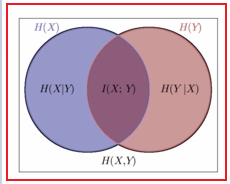相对熵（relative entropy），又被称为Kullback-Leibler散度（Kullback-Leibler divergence）或信息散度（information divergence），是两个概率分布（probability distribution）间差异的非对称性度量。在信息理论中，相对熵等价于两个概率分布的信息熵（Shannon entropy）的差值 。相对熵表示使用理论分布拟合真实分布时产生的信息损耗。

定义：设 $P(x)$, $Q(x)$ 是随机变量 $X$ 上的两个概率分布，则在离散和连续随机变量的情形下，相对熵的定义分别为:

$\begin{array}{l} \mathrm{KL}(P \| Q)=\sum P(x) \log \frac{P(x)}{Q(x)} \\ \mathrm{KL}(P \| Q)=\int P(x) \log \frac{P(x)}{Q(x)} d x \end{array}$

与信息理论中其它概念的关系：对前向KL散度，其值等于真实分布与拟合分布的交叉熵与真实分布的信息熵之差：

$\mathrm{KL}(P \| Q)=H(P, Q)-H(P)$

## 3.3 拓扑正相关抽样(TAPS)

获得的邻居表示 $\overrightarrow{\boldsymbol{s}}_{i}^{(l)}$ ，所存在的问题是：

• [li]首先，整个社区可能包含冗余甚至有噪声的信息。
• 其次，聚合操作的计算代价高昂。
[/li]

为解决这个问题，本文提出 TAPS 策略。

对于一个节点 $v_{i}$，使用 $X_{i}$ 代表它的拓扑信息。$X_{i}$ 可以代表其邻域 $\mathcal{N}_{i}$ 所提供的信息量，也可以代表着其非邻域 $\overline{\mathcal{N}_{i}}=\mathcal{V}-\mathcal{N}_{i}$ 所带来的信息。基于$X_{i}$，我们定义 $p\left(X_{i}=\mathcal{N}_{i}\right)=\frac{\left|\mathcal{N}_{i}\right|}{|\mathcal{V}|}$ 和 $p\left(X_{i}=\overline{\mathcal{N}_{i}}\right)=\frac{\left|\mathcal{V}-\mathcal{N}_{i}\right|}{|\mathcal{V}|}$ ，其中 $|\cdot|$ 代表着基函数（可增可减）。$p\left(X_{i}=\mathcal{N}_{i}\right)$ 表示当我们在图上随机采样一个节点时，该节点落入 $v_{i}$ 邻域的概率。此外，对于相邻的两个节点 $v_i$ 和 $v_j$，我们可以定义以下联合概率：

$\begin{array}{l} p\left(X_{i}=\mathcal{N}_{i}, X_{j}=\mathcal{N}_{j}\right)=\frac{\left|\mathcal{N}_{i} \cap \mathcal{N}_{j}\right|}{|\mathcal{V}|} \\ p\left(X_{i}=\mathcal{N}_{i}, X_{j}=\overline{\mathcal{N}_{j}}\right)=\frac{\left|\mathcal{N}_{i} \cap\left(\mathcal{V}-\mathcal{N}_{j}\right)\right|}{|\mathcal{V}|} \\ p\left(X_{i}=\overline{\mathcal{N}_{i}}, X_{j}=\mathcal{N}_{j}\right)=\frac{\left|\left(\mathcal{V}-\mathcal{N}_{i}\right) \cap \mathcal{N}_{j}\right|}{|\mathcal{V}|} \\ p\left(X_{i}=\overline{\mathcal{N}_{i}}, X_{j}=\overline{\mathcal{N}_{j}}\right)=\frac{\left|\left(\mathcal{V}-\mathcal{N}_{i}\right) \cap\left(\mathcal{V}-\mathcal{N}_{j}\right)\right|}{|\mathcal{V}|}, \end{array}\quad\quad\quad(7)$

其中 $p\left(X_{i}=\mathcal{N}_{i}, X_{j}=\mathcal{N}_{j}\right)$ 是随机选择的节点落入 $v_i$ 和 $v_j$ 相交邻居的概率。基于互信息，我们将 $v_i$ 和 $v_j$ 之间的图结构依赖关系定义为：

\begin{aligned}I\left(X_{i} ; X_{j}\right)=& \sum\limits _{X_{i}} \sum\limits_{X_{j}} p\left(X_{i}, X_{j}\right) \cdot \log \frac{p\left(X_{i}, X_{j}\right)}{p\left(X_{i}\right) \cdot p\left(X_{j}\right)} \\ & \text { s.t. } v_{j} \in \mathcal{N}_{i} . \end{aligned}\quad\quad\quad\quad(8)

上面的图结构依赖值主要度量两个节点的拓扑相似性。值越大，表示两个节点之间有很强的依赖性。

在我们的 TAPS 策略中，我们通过排序选择和 $v_i$ 正相关依赖值的邻居节点，然后通过 Aggregator 操作获得节点$v_i$的邻居节点表示 $\overrightarrow{\boldsymbol{s}}_{i}^{(l)}$。当只选择一个正节点时，我们直接选择对 $v_i$ 依赖值最大的节点 $v_j$，从而避免了昂贵的聚合操作。同时，由于图的拓扑结构仅依赖于邻接矩阵，TAPS允许我们预先进行正采样，这可以避免训练过程中的正采样开销。

# 4 训练框架

有三种基于图的自监督训练方案。

• [li]第一种类型是 Pre-training 和 Fine-tuning（PT和FT）。预训练阶段首先用代理任务初始化GNN编码器的参数。在此之后，这个预先训练好的GNN编码器将在特定的下游任务的监督下进行微调。
• 第二种是 Joint Learning (JL) 方案，其中GNN编码器、代理任务和下游任务被联合训练。
• 最后一种类型是无监督表示学习(URL)。与 PT&FT 类似，URL也遵循了一个两阶段的训练方案，其中第一阶段基于代理任务对GNN编码器进行训练，但在第二个下游任务阶段，GNN编码器被冻结。
[/li]

在我们的工作中，我们同时采用JL和URL管道来训练和评估我们的网络。

## 4.1 JL 训练框架

如 Figure 1.(a)说明了 JL 训练过程。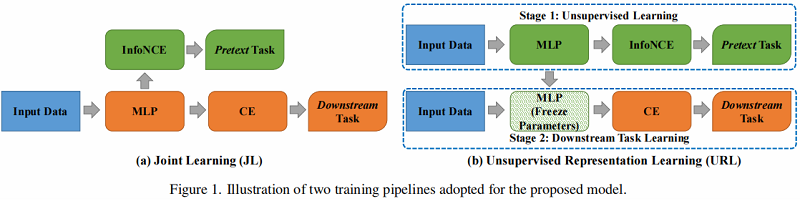可以看出，不像大多数现有的基于图的SSL工作，使用GNN作为节点/图编码器，我们简单地使用一个浅的MLP作为编码器，这更有效。在JL方案中，我们在MLP编码器输出的节点表示之上联合应用InfoNCE损失和交叉熵损失：

$\mathcal{L}=(1-\alpha) \mathcal{L}_{\mathrm{CE}}+\alpha \mathcal{L}_{\mathrm{InfoNCE}}\quad\quad\quad(9)$

## 4.2 URL 训练框架

如 Figure 1.(b) 所示，包括两个训练阶段：训练前的代理任务使用 InfoNCE 损失 $\mathcal{L}_{\mathrm{InfoNCE}}$ 训练 MLP 编码器，下游任务使用交叉熵损失 $\mathcal{L}_{\mathrm{CE}}$ 学习线性节点分类器。

# 5 实验

## 5.1 实验设置

6 个节点分类数据集：Cora，Pubmed，citsee，Amazon Photo，Coauthor CS  和 Coauthor Physics 。

## 5.2 基线

对比的方法：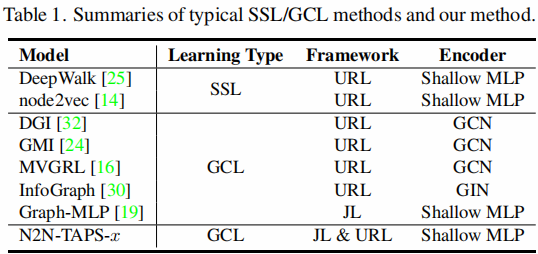## 5.3 结果分析

Table 2 显示了本文的方法与其他选择的方法之间的性能比较。• 我们的 N2N 模型，无论是 N2N(JL) 还是 N2N(URL)，在所有 6 个数据集上的表现始终优于比较方法。在 Cora、Pubmed 和 Coauthor CS 等数据集上，提高率可以高达 3%。这表明了 N2N 互信息最大化策略在 GNN 和其他基于 GCL 的节点表示学习方面的竞争力。另一个值得一提的问题是，由于我们基于 N2N 的方法避免了拓扑增强，而是简单地使用 MLP 作为节点编码器，我们的方法在训练和推理方面更有效。
• 在 N2N 系列中，我们在基于 TAPS 抽样更多的正相关邻居，通常观察到改善，但改善是有限的。这表明了 N2N-TAPS-1 的潜力，因为它避免了已知的昂贵的邻域聚合操作。然而，当从附近随机抽样单个正样本时，性能显著下降。这一结果表明，所提出的战术策略确实可以采样拓扑意义的正相关邻居。
• 在现有的方法中，GCL 方案与有监督的 GNN 变体相比，具有类似的性能，甚至稍微更好的性能。这一观察结果表明，SSL 可能是基于图的表示学习中的一种很有前途的替代方法。

# 5.4 消融实验

### 5.4.1 基于随机正抽样的 N2N(JL)

在本节中，基于随机正抽样的 N2N(JL)。为了进一步证明我们的 TAPS 策略的必要性和优势，通过将采样量从 $1$ 改变到 $5$ 来进行随机正抽样实验。我们选择了两个数据集，即Amazon Photo 和 Coauthor Physics 来做这个实验，因为它们的 平均节点度>5。对于每个采样量，我们用不同的随机种子进行了三次实验。结果如 Table 3 所示。从表中我们可以清楚地观察到，随机正抽样导致较大的性能方差，这意味着随机抽样不能识别一致的和信息丰富的邻居。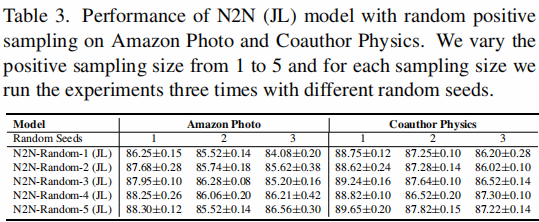### 5.4.2 时间消耗对比

我们的方法被期望比现有的工作更有效。一方面，我们的工作采用MLP作为节点编码器，从而避免了编码阶段昂贵的节点聚合。另一方面，陷阱使我们能够预先取样有限的高质量阳性。特别是，当选择了一个正相关样本时，我们就完全摆脱了聚合操作。

Table 4 显示了时间消耗的比较。从结果中我们可以看到，我们的方法可以比典型的基于 GNN 和 GCL 的方法快几级。GraphMLP  也采用了MLP作为编码器，但它使用的是所有邻居节点信息，这就解释了它在 CS 和 Physics 等大型数据集上的缓慢性。### 5.4.3 TAPS策略评估

TAPS 是我们框架中保证正采样质量和效率的重要组成部分。在 Table 2 中，我们展示了 N2NTAPS-1 由于基于 TAPS 随机抽样的优势。在本节中，我们将TAPS采样应用于另一个基于 GNN 基线 GraphSAGE-Mean，以验证 TAPS 是否可以作为一般的邻域采样策略来识别信息邻域。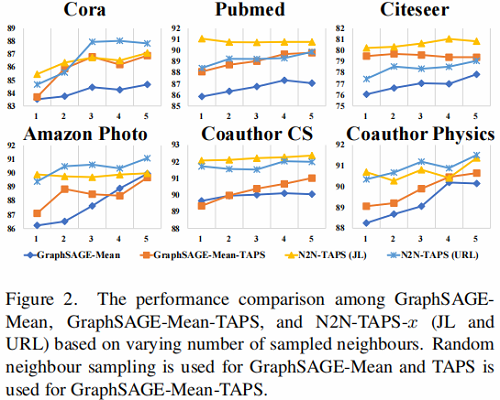结果如 Fig.2 所示。默认情况下，GraphSAGE-Mean 使用随机抽样来选择邻居进行聚合，这有接收噪声信息的风险。我们用 TAPS 替换 GraphSAGE-Mean中的随机抽样，并保持所有其他实现的完整。它的性能明显得到了提高，通常使用更多的邻居可以更有利于性能。这个观察告诉我们，考虑结构依赖关系来选择有用的邻居来丰富节点表示是很重要的。

### 5.4.4 标签平滑性分析

为了利用 TAPS 策略验证邻域采样的质量，我们引入了 CSGNN 中提出的标签平滑度度量

$\delta_{l}=\sum_{\left(v_{i}, v_{j}\right) \in \mathcal{E}}\left(1-\mathbb{I}\left(v_{i} \simeq v_{j}\right)\right) /|\mathcal{E}|$

其中：$\mathbb{I}(\cdot)$ 是一个指示函数，即当 $y_{v_{i}}=y_{v_{j}}$ 时 $\mathbb{I}\left(v_{i} \simeq v_{j}\right)=1$，当 $y_{v_{i}} \neq y_{v_{j}}$ 时，$\mathbb{I}\left(v_{i} \simeq v_{j}\right)=0$。

一个大的 $\delta_{l}$ 表明具有不同标签的节点被认为是连接的邻居，而一个较小的 $\delta_{l}$ 表示一个具有更高质量的邻域结构的图 $\mathcal{G}$，即一个节点的大多数邻域与该节点具有相同的标签。拥有小 $\delta_{l}$  即代表了高质量的邻域，这可以为其相应的中心节点提供同质的信息增益。

Fig.3 显示，通过我们的 TAPS 策略将采样量从 $1$ 扩大到 $5$，标签平滑值逐渐增加。在没有任何采样策略的情况下，整个图的标签平滑度值最高。这一现象表明，我们的 TAPS 策略可以提高邻域采样质量，这解释了为什么所提出的 N2N-TAPS-1 模型在某些数据集上具有竞争性能。

TAPS 策略本质上是一种子图划分方案。一个好的子图内部节点标签应该一致度高。Figure 4 显示了子图的大小（子图中的节点）和由TAPS得到的这些子图的数量方面的统计分布。Cora 上的子图划分的细节如 Fig.5 所示，其中不同的节点颜色代表不同的标签。在每个子图中，大多数节点都有相同的颜色（相同的标签），甚至在一些大型的子图中，这意味着 TPAS 生成了高质量的邻域。这种可视化还揭示了我们的TAPS策略能够在图中建模多跳上下文信息，尽管我们没有明确地这样做。其他数据集的统计分布和子图划分的详细信息见附录B和C。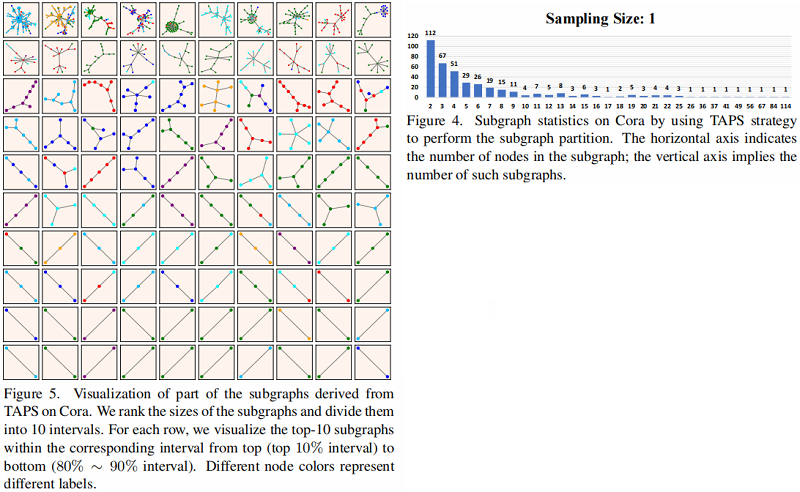# 6 结论

本文提出了一种简单而有效的自监督节点表示学习策略，通过互信息最大化，直接优化节点的隐藏表示与其邻域之间的对齐。理论上，我们的公式鼓励图形平滑。我们还提出了一个战术策略来识别信息丰富的邻居，并提高我们的框架的效率。值得一提的是，当只选择一个正节点时，我们的模型可以完全避免邻域聚合，但仍然保持着良好的节点分类性能。一项有趣的工作将是将提出的自监督节点表示学习和邻域采样策略扩展到异构图数据。

# 参考论文

 Principal Neighbourhood Aggregation for Graph Nets.关于 Aggregation 中 ensemble 方法：
 Inductive representation learning on large graphs.
 Graph attention networks.
 On the efficacy of knowledge distillation.
 Mutual information neural estimation.
 Measuring and improving the use of graph information in graph neural networks.
 Graph self-supervised learning: A survey.
 Relational knowledge distillation.